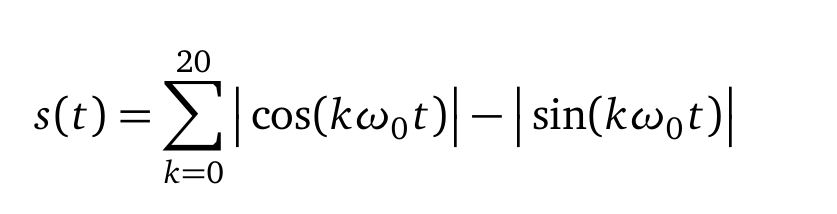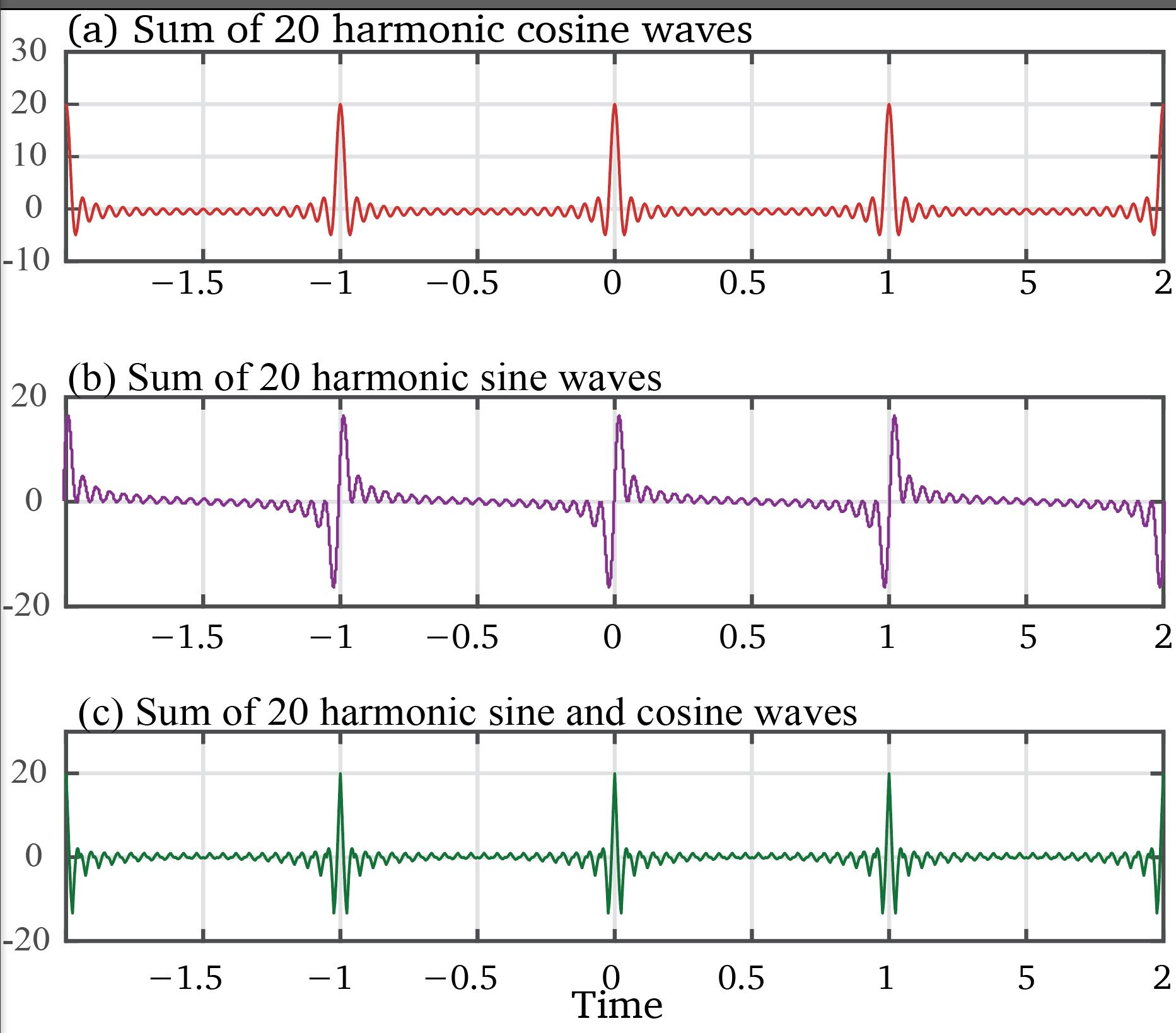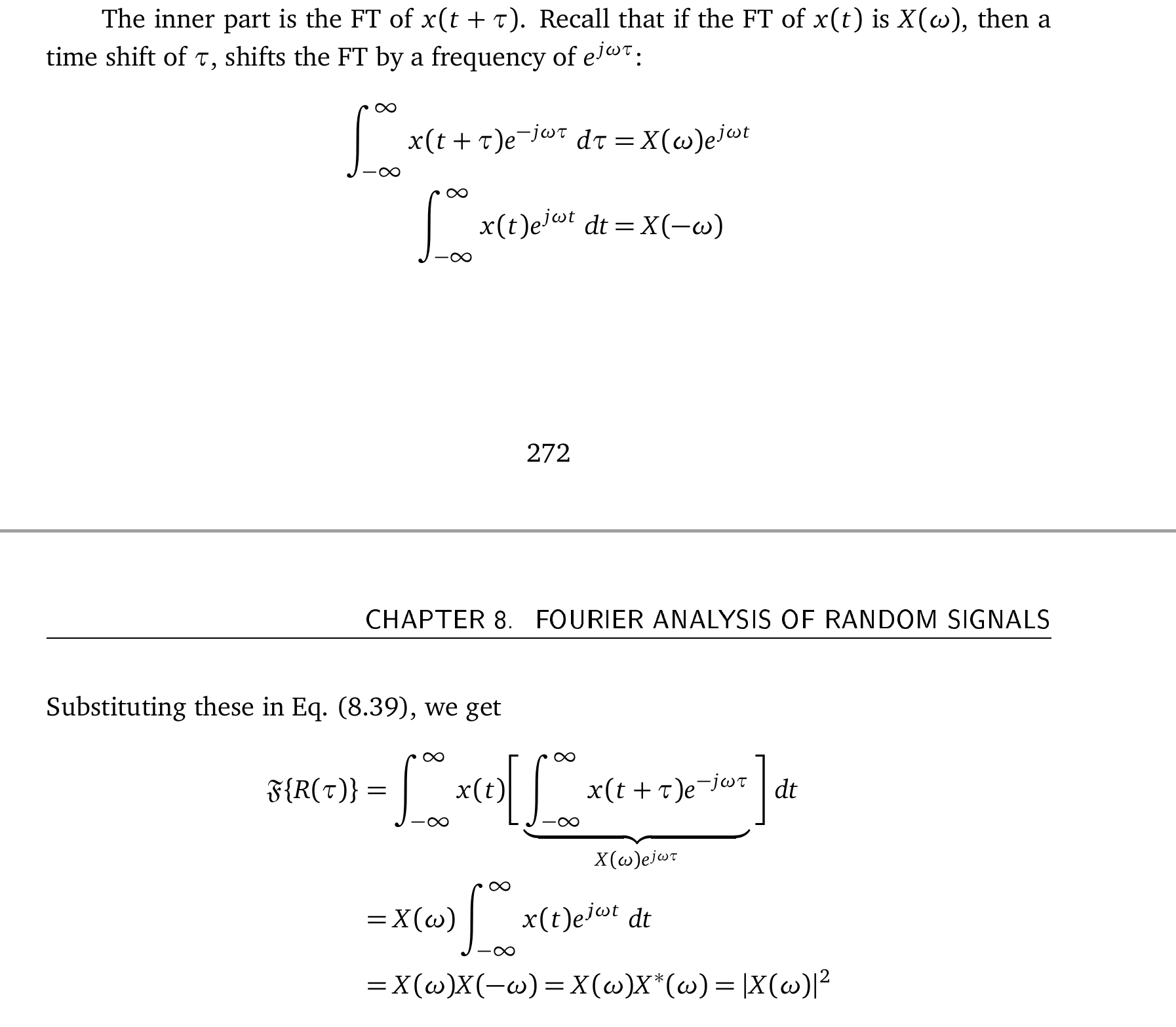# Intuitive Guide to Fourier Analysis .. Errata sheet

#### Errata List for “Intuitive Gide to Fourier Analysis and Spectral Estimation”, Second printing

Errata, book 2nd printing – for printed copy only.
Kindle book has been corrected for all of these.

• Chapter 1- Fig. 1.10.c The equation on page 14 for this graph should be to index of 20, as follows.
•Here I was trying to show that the summation of a bunch of sinusoids approaches a delta function, but that we can only do with the sines with a negative sign in front as  above. The plot in 1.10c then changes and looks more like what we want to see in (c).

• Brought to my attention by John John Steigerwalt – Thanks.
•• Division by T is not needed in Equation 3.8.
• On page 79, in the code snippet for a sinc function “habs = abs(h1)”, there is no variable “h1” defined. It should be “h”.
• In equation 3.34, the power multiplier “k” for the first exponential is not needed
• On page 98, to describe equation 3.38 should refer to 2.24.
• In example 3.10 on page 103, the sigma symbol is missing in the formulas.
• In example 3.11 on page 104, for C1, C2, C3, the formulas are missing 1/4 multiplier and the sum should loop over “n” instead of “k”.
• In equation 4.22 (2nd row), “j” has already been moved out of the sin expression. “j” squared is not needed inside the sin expression.
•  In example 4.5, the power “-1” is missing to indicate that it is inverse transform for x(t) expression.
•  In equation 4.32, for  X(w) for the Gaussian, move 1/2 multiplier out of the integral.
• In equation 4.34, argument to sinc function should not be divided by PI.
•  On page 137, the formula for x(t) and the computations based on x(t) are missing “k” in the power of the complex exponential. It is correctly used in equation 4.35.
•  In example 5.2, bottom of the page, “imaginary” part should have “+” before the second sin parameter.
•  In example 5.4, reference to figure 5.7 (at the top of page 158), should be figure 5.11.
•  Equation 6.3 is missing minus sign in the power of the complex exponential.
• In a comment to figure 6.5, it is said “DTFT is shown as a dashed line for reference”. The DTFT is shown as a solid blue line.
•  Chapter 8: Eq. 8.39 changed as follows.• 17. at the bottom page 24, the equation for the target signal with two harmonics it missing a “t” from the term “b_3 sin(3*omega)”___________________________________________________________

For the older book – this book no longer available. Amazon and the Kindle version are new one.

##### Errata List for “Intuitive Gide to Fourier Analysis and Spectral Estimation”, First Edition

Feel embarrassed that after reading the stuff more than twenty times, I did not notice so many glaring errors. Well, that’s my excuse any way! Your indulgence required until the next edition.

Here I have listed all the errors and typos that have already been corrected in the Kindle version but remain in the First edition of the book. Please add your discoveries below in the comment section. .

These apply only to the printed book. They have already been corrected in the electronic version.

(Equations are given in Latex)

\item Chapter 1: page 21, below Eq. 1.16, the last line should say {Now the whole expression uses only the cosine waves and the index, $k$ spans not just from $0$ to $\infty$, but from $-\infty$ to $+\infty$.}

\item Chapter 1: page 17, Fig. 1.13(b and d) the sign for the second term should be negative, i.e. $-{1}{3}\cos(3 \omega_0 t)$ $-{1}{7}\cos(3 \omega_0 t)$.

\item Chapter 2: Eq. 2.11 thru Eq. 2.22 should have a subscript 0 to the frequency, $\omega_0$ instead of $\omega$ alone. This subscript makes it clear that $\omega$ is not a variable but is a constant, the starting or the fundamental frequency.

\item Chapter 2: Eq. 2.21 and in Eq. 2.22 the sign of the exponential is reversed. The first one should be minus and second, a plus.

\item Chapter 2: Eq. 2.24 is missing the term $t$, should be $e^{\j k \omega \underline{t}}$.

\item Page 59: The duty cycle term is $\tau{/T}$ and not $\tau$ alone.

\item Page 57: Example 2.9, the signal should be \newline
$x(t) = 3+ 6\cos(4\pi t+2)+\underline{4}\j\sin(4\pi t+3)- 3\j \sin(10\pi t+1.5)$
\newline Note the underlined quantity $4$ for the third term, $\j \sin(4\pi t+3)$.

\item Page 58: The calculated value of the coefficient $e^{-\j 4\pi t}$ should be 2.552. The corresponding Fig. 2.14 has been changed.

\item Page 68: Example 2.15 The correct answer is -0.142 instead of 0.142.

\item Page 74: Figure 3.3 (a), the y axis has been moved to the center of the shape to be consistent with figures (b) and (c).

\item Page 75: Eq. 3.8, added subscript s to Time T.

\item Page 83: Last line, the equation number should be (3.17) instead of (3.19).

\item Page 86: First paragraph, last line, should be “with only 1 Hz” and not 2 Hz.

\item Page 92: Third paragraph, third line, should be $11 \pi /5$ and not $12 \pi /5$

\item Page 97: Eq. (3.37) and eq. (3.38) et. all are missing the coefficient $1/N_0$ from the front of the RHS.

\item Page 102: The correct values for the coefficients are: 1.5, .612, 0.5 and 0.612, as shown in the figure 3.25.

\item Page 103: Correct coefficients are: 0.75, 0.56, 0.25 and 0.56.

\item Page 104: Added subscript 0 to N to clarify that this is the fundamental period.

\item Page 149: N is equal to ${(2M+1)}$ and not $\frac{(2M+1)} {2}$.

\item Page 157: Eq.(5.22) is missing the coefficient $\frac{1}{N_0}$ in the front.

\item Page 190: The index of the top equation should be 6 and not 7.

### 19 Comments on “Intuitive Guide to Fourier Analysis .. Errata sheet”

1.Chandru Aswani says:

In Chapter 2, Eq 2.3, I noticed that the right-hand side contains a ‘j’ in the denominator. Is this a typo?

Many thanks for this lucid book!

•Charan L. says:

Do believe you are correct. the “j” on the numerator should not be there. Another item to be added to the erratta. Thanks for pointing it out. How do you like the book otherwise?
Charan Langton Please send me your address, I will send you a free copy of the book.

2.Tarun Cousik says:

Hi Charan,

I recently got your book(digital copy) and I’m planning to plow through it over the course of this month. I love the fact that you made this page for correcting errors, wish other authors would do the same :). If I find any errors, I’ll keep you posted here.

My first one- small error, but it had me scratching my head for 5 mins. Page 17, Fig 1.13b and Fig 1.13d, I believe there should be a minus sign before the (1/3)cos(3wt) and (1/7)cos(7wt) terms in the figure’s description. You stated it correctly in the equations below (eqns. 1.9, that’s where I conformed my suspicion).

3.Pascal Renucci says:

Hi Charan,

I’ve been a big fan for many years, as yours was one of the first DSP/comms-explained sites, which I found during my stint at Lockheed in Sunnyvale, CA, working on the MUOS program.

I was thrilled to see recently the publication of this book, and immediately bought it. The book is a goldmine and I plan on reading it cover-to-cover. The typos are inevitable, don’t worry.

I did find one, I believe, just now: bottom of page 68 purports to list an identity for |z| = 0.5*(z + z*). However, the RHS yields Re{z}, not |z|.

4.Zhe.zhu says:

Hi Charan：

I have find a same error as Tarun Cousik did, chapter 1, Figure 1.13, page 13. it should be “x2 = x1 – (1/3)cos(3wt) and x4 = x3 – (1/7)cos(7wt)” but your are ” x2 = x1 + (1/3)cos(3wt) and x4 = x3 + (1/7)cos(7wt) ” . And, by the way, for sine waves i.e. Figure 1.12, the last summed wave are presented with “x(t)”, but in your cosine waves i.e. Figure 1.13,page 13, the last summed wave are expressed with x4.

Have good day!

Zhe.zhu

5.varun says:

Here is link to the errata pdf to the book I made in Latex online

6.Charan L. says:

Thank you all. I do think the second printing is a lot better.

7.mark leeds says:

Hi Charan: I am a DSP beginner and I have the first printing of the book. I just started looking at it and I’m very scared of typos because of my shallow DSP knowledge. Is it possible to exchange the first printing of the text for a second printing ? Thanks.

8.Carlos Anzuela says:

Hi Charan:

I think in the Figure 4.14(c) the X(w) picture is wrong. The triangular pulse has the depicted Sinc^2 expression, but the picture of X(w) is different. It looks like a mistake in the copy/paste of the figure from de above 4.14(b) Sinc figure. I would like to attach a picture but I do not know how to do it. Anyway congratulations for you book and for your web site, that make easy the complex concepts in communications.
X(w)
_|_
/ | \
__ / | \ __
__ / \ / | \ / \ __
___/__\___/____\___/____|____\___/____\___/__\____ w

9.james says:

Hi Charan：
is there something wrongs on page 123 at eq4.14(e^-jwt), 4.15(1/2pi), 4.16(e^-jw(t-t0)), 4.17(1/2pi) ?

sincerely
James

•Charan L. says:

HI James, I will look into this.
Otherwise, are you getting what you were hoping from the book?
Charan

10.Mykola says:

Hey Charan,

I really enjoy reading your book! I think I might have spotted a typo in FFTBookSample.pdf in a formula at the bottom of the page 21.

The 2nd sine harmonic seems to be missing argument “t”.

Thanks!

11.Srinivas K says:

Chapter 2 Appendix.
Example 2.12. Convert z = −1 − j to polar form M = Æ (−1) 2 + (−1) 2 = p 2 θ = arctan y x + π, since x < 0 = arctan −1 −1 + π = 3 4 π ⇒ z = p 2∠ 3 4 π.

The answer for the angle stated as 3(pi)/4 is incorrect. It should be 5(pi)/4 (225 deg). When you add something (in this case pi/4 radians) to pi radians (180 deg), the result cannot be less than pi radians (180 deg).

•Charan L. says:

Thanks. I will fix it.

•Charan L. says:

Srinivas, Check it in Matlab
s = -1 -j;
angle(s)=2.3562
abs(s) = 1.414
(3/4)*pi = 2.3562

12.Alessandro Bertulli says:

Hi! Thanks for this work, I really like it, and I am finding here all the “ah-ha!” moments I missed from other resources.

I am looking at the sample of Chapter 2 from the blog (https://complextoreal.com/tutorials/tutorial-5-fourier-analysis-made-easy-part-2/). In page 59, example 2.10, I read “Replacing the duty cycle term τ with term r, the equation becomes easier to understand.”. Shouldn’t it be: “Replacing the duty cycle term τ/T with term r, the equation becomes easier to understand.”, that is, the duty cycle should be τ/T ?

Thanks again.

13.Alessandro Bertulli says:

In Example 3.10, at page 101, it is said “the values of the complex exponential for argument
π/4 are 0 and 1 respectively for the cosine and sine”. Of course, it should have been π/2.

This site uses Akismet to reduce spam. Learn how your comment data is processed.